## Tuesday, December 16, 2008

### Irodov Problem 1.259Motion of mass m: There are two forces acting on mass m, i) the force of gravity mg and ii) the tension in the string T1. Let its acceleration be a1. So from Newton's laws we have,

Motion of mass M: There are four forces acting on mass M, i) the force of gravity Mg, ii) the tension in the string connecting to mass m i.e. T2, iii) the forces on the two strings holding mass m of T1 each. Let the acceleration of mass M be a2. So we have,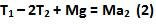Since the force of gravity Mg passes through the axis of rotation of mass M, it does not produce any torque in it. However, the rest of the three forces produce torques inducing rotation in the mass. Since the tensions T2 act at a distance of R from the axis, they produce a torque of T2R each in the clockwise direction. The tension T1 acts at a distance of 2R from the axis of rotation of mass M and so it induces a torque of T1(2R) on the mass also in the clockwise direction. Let the angular acceleration of mass M be. Then we have,

Since the threads holding mass M do not slip we have,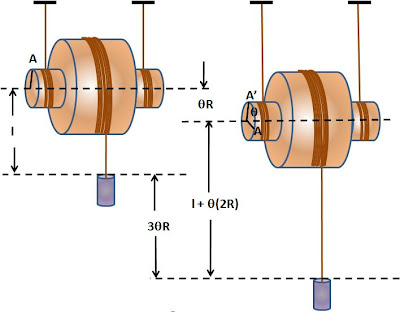As seen in the figure, suppose that the mass M rotates an angle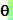, each of the two threads unwrap a length ofand so the mass M falls down this distance. Meanwhile, the rotation of mass M also releases a length of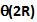of the thread holding mass m, making the distance between mass M and mass m to increase by this additional distance. Consequently the total distance moved by mass m is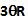as shown in the figure. From this discussion and (4) it is clear that,Now using (1), (2), (5) and (6) we can solve for a1 as follows,Anonymous said...

PLEASE EXPLAIN THE USE OF 2THETA R

Krishna Kant Chintalapudi said...

The thicker part of the spindle has a radius of 2R, so for the same angular rotation theta, 2(theta)R rolls out.sumilak said...

the solutions are really helpful in cases of such tough problems